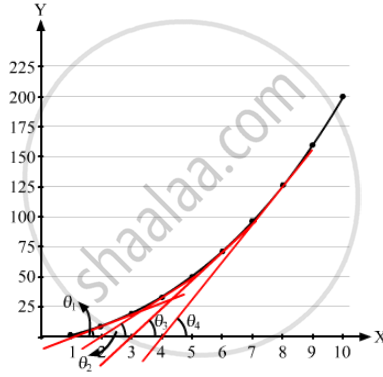# Draw a Graph from the Following Data. Draw Tangents at X = 2, 6 and 8. Find the Slopes of These Tangents. Verify that the Curve Draw is - Physics

Sum

Draw a graph from the following data. Draw tangents at x = 2, 4, 6 and 8. Find the slopes of these tangents. Verify that the curve draw is y = 2x2 and the slope of tangent is $\tan \theta = \frac{dy}{dx} = 4x$
$\begin{array}x & 1 & 2 & 3 & 4 & 5 & 6 & 7 & 8 & 9 & 10 \\ y & 2 & 8 & 18 & 32 & 50 & 72 & 98 & 128 & 162 & 200\end{array}$

#### Solution

Note: Students should draw the graph y = 2x2 on a graph paper for results.

To find a slope at any point, draw a tangent at the point and extend the line to meet the x-axis. Then find tan θ as shown in the figure.The above can be checked as follows:

$Slope = \tan \theta = \frac{dy}{dx}$

$= \frac{d}{dx}\left( 2 x^2 \right) = 4x$
Here, x = x-coordinate of the point where the slope is to be measured.

Concept: What is Physics?
Is there an error in this question or solution?
Chapter 2: Physics and Mathematics - Exercise [Page 29]

#### APPEARS IN

HC Verma Class 11, Class 12 Concepts of Physics Vol. 1
Chapter 2 Physics and Mathematics
Exercise | Q 20 | Page 29
Share